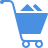Cart (0)

In a linear model, the relationship between the dependent variable (e.g., sales) and independent variable (e.g., advertising expenditures) is considered linear. If the relationship between sales and advertising expenditures is 1:5, it assumes that it will remain the same for every level of advertising expenditures. That is, when advertising expenditures increase by one dollar, sales will increase by 5 units, if it increases by two dollars, sales will increase by 10 units, and so on.

Previous Entry
Next Entry